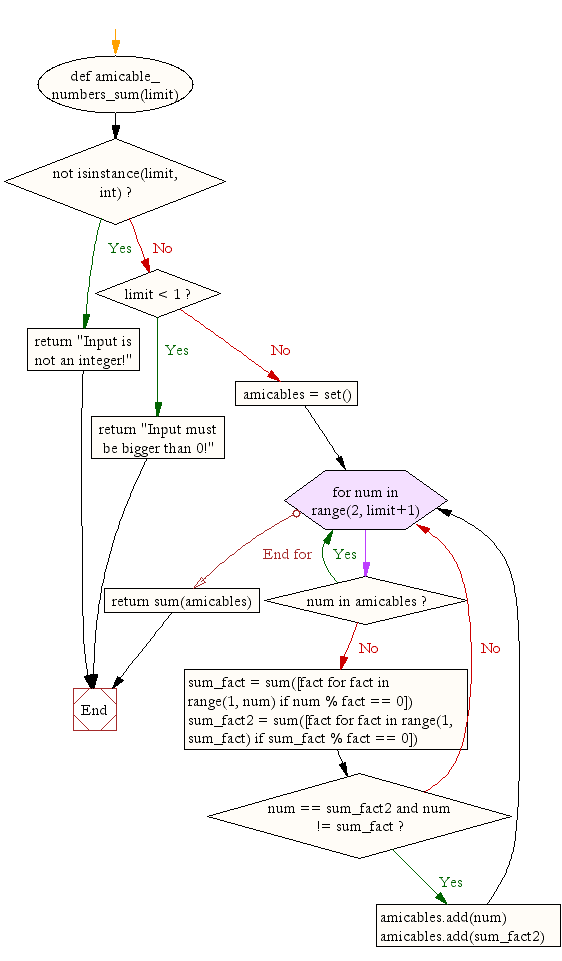﻿ Python Math: Sum all amicable numbers from 1 to specified numbers - w3resource# Python Math: Sum all amicable numbers from 1 to specified numbers

## Python Math: Exercise-14 with Solution

Write a Python program to sum all amicable numbers from 1 to specified numbers.
Note: Amicable numbers are two different numbers so related that the sum of the proper divisors of each is equal to the other number. (A proper divisor of a number is a positive factor of that number other than the number itself. For example, the proper divisors of 6 are 1, 2, and 3.)

Sample Solution :-

Python Code :

``````def amicable_numbers_sum(limit):
if not isinstance(limit, int):
return "Input is not an integer!"

if limit < 1:
return "Input must be bigger than 0!"

amicables = set()

for num in range(2, limit+1):
if num in amicables:
continue

sum_fact = sum([fact for fact in range(1, num) if num % fact == 0])
sum_fact2 = sum([fact for fact in range(1, sum_fact) if sum_fact % fact == 0])
if num == sum_fact2 and num != sum_fact:

return sum(amicables)

print(amicable_numbers_sum(9999))
print(amicable_numbers_sum(999))
print(amicable_numbers_sum(99))
```
```

Sample Output:

```31626
504
0
```

Pictorial Presentation:Flowchart:## Visualize Python code execution:

The following tool visualize what the computer is doing step-by-step as it executes the said program:

Python Code Editor:

Have another way to solve this solution? Contribute your code (and comments) through Disqus.

What is the difficulty level of this exercise?

Test your Programming skills with w3resource's quiz.

﻿

```>>> students = [{'name': 'John', 'score': 98}, {'name': 'Mike', 'score': 94}, {'name': 'Jennifer', 'score': 99}]